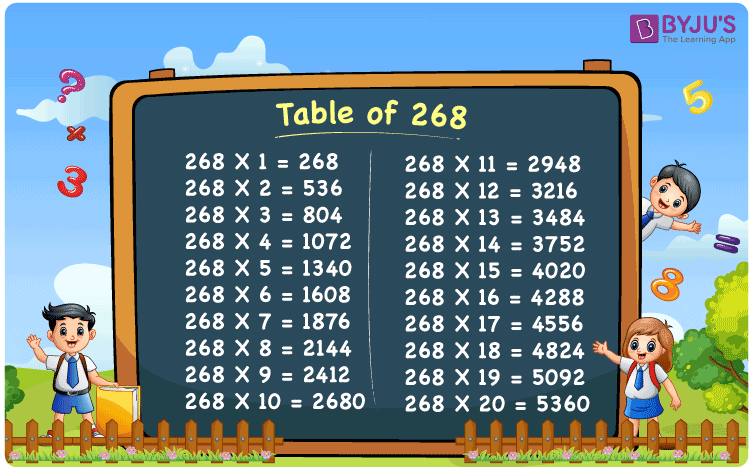Checkout JEE MAINS 2022 Question Paper Analysis : Checkout JEE MAINS 2022 Question Paper Analysis :

# Table of 268

The table of 268 is the multiplication table, which is the result of the product of 268 with the natural numbers. Learning 268 times is important, as it helps to solve mathematical problems quickly. In this article, we have provided the multiplication table of 268 in tabular form, and a 268 times chart. Download the PDF for the table of 268, by clicking the link below.

## Table of 268 Chart## What is the 268 Times Table?

The 268 times table is the table of 268 which gives the repeated addition of 268 for the specified number of times. For example, 268 × 3 = 804.

It can also be written as 268 + 268 + 268 = 804.

The table of 268 using the repeated addition and multiplication methods is given here up to the first 10 times.

 268 × 1 = 268 268 268 × 2 = 536 268 + 268 = 536 268 × 3 = 804 268 + 268 + 268 = 804 268 × 4 = 1072 268 + 268 + 268 + 268 = 1072 268 × 5 = 1340 268 + 268 + 268 + 268 + 268 = 1340 268 × 6 = 1608 268 + 268 + 268 + 268 + 268 + 268 = 1608 268 × 7 = 1876 268 + 268 + 268 + 268 + 268 + 268 + 268 = 1876 268 × 8 = 2144 268 + 268 + 268 + 268 + 268 + 268 + 268 + 268 = 2144 268 × 9 = 2412 268 + 268 + 268 + 268 + 268 + 268 + 268 + 268 + 268 = 2412 268 × 10 = 2680 268 + 268 + 268 + 268 + 268 + 268 + 268 + 268 + 268 + 268 = 2680

## Multiplication Table of 268

The table of 268 for the first 20 natural numbers is given here.

 268 × 1 = 268 268 × 2 = 536 268 × 3 = 804 268 × 4 = 1072 268 × 5 = 1340 268 × 6 = 1608 268 × 7 = 1876 268 × 8 = 2144 268 × 9 = 2412 268 × 10 = 2680 268 × 11 = 2948 268 × 12 = 3216 268 × 13 = 3484 268 × 14 = 3752 268 × 15 = 4020 268 × 16 = 4288 268 × 17 = 4556 268 × 18 = 4824 268 × 19 = 5092 268 × 20 = 5360

## Solved Example on the Table of 268

Example:

If a box contains 268 oranges, how many oranges are in 8 such boxes?

Solution:

Given that, 1 box = 268 oranges.

To find the number of oranges in 8 boxes, we have to multiply 8 by 268.

I.e., 8 × 268 = 2144.

Therefore, there are 2144 oranges in 8 boxes.

## Frequently Asked Questions on the Table of 268

### What is the table of 268?

The table of 268 is the multiplication table, which gives the result as the multiples of 268.

### Write the 268 times table up to 10 times.

268 × 1 = 268, 268 × 2 = 536, 268 × 3 = 804, 268 × 4 = 1072, 268 × 5 = 1340, 268 × 6 = 1608, 268 × 7 = 1876, 268 × 8 = 2144, 268 × 9 = 2412, 268 × 10 = 2680.

### What is 268 times 20?

268 times 20 is 5360. I.e., 268 × 20 = 5360.